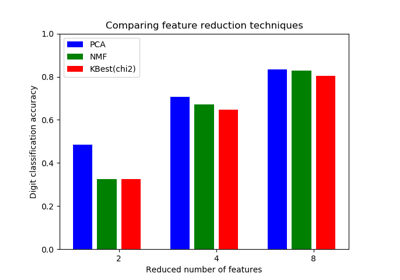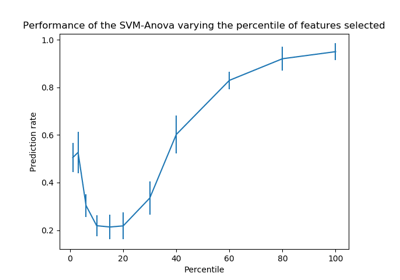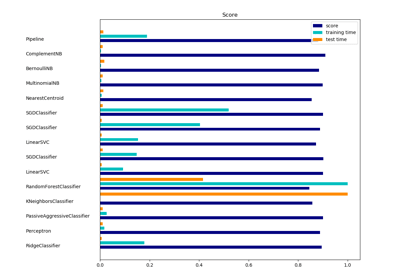/scikit-learn

# sklearn.feature_selection.chi2

`sklearn.feature_selection.chi2(X, y)` [source]

Compute chi-squared stats between each non-negative feature and class.

This score can be used to select the n_features features with the highest values for the test chi-squared statistic from X, which must contain only non-negative features such as booleans or frequencies (e.g., term counts in document classification), relative to the classes.

Recall that the chi-square test measures dependence between stochastic variables, so using this function “weeds out” the features that are the most likely to be independent of class and therefore irrelevant for classification.

Read more in the User Guide.

Parameters: `X : {array-like, sparse matrix}, shape = (n_samples, n_features_in)` Sample vectors. `y : array-like, shape = (n_samples,)` Target vector (class labels). `chi2 : array, shape = (n_features,)` chi2 statistics of each feature. `pval : array, shape = (n_features,)` p-values of each feature.

`f_classif`
ANOVA F-value between label/feature for classification tasks.
`f_regression`
F-value between label/feature for regression tasks.

#### Notes

Complexity of this algorithm is O(n_classes * n_features).

## Examples using `sklearn.feature_selection.chi2`Selecting dimensionality reduction with Pipeline and GridSearchCVSVM-Anova: SVM with univariate feature selectionClassification of text documents using sparse features